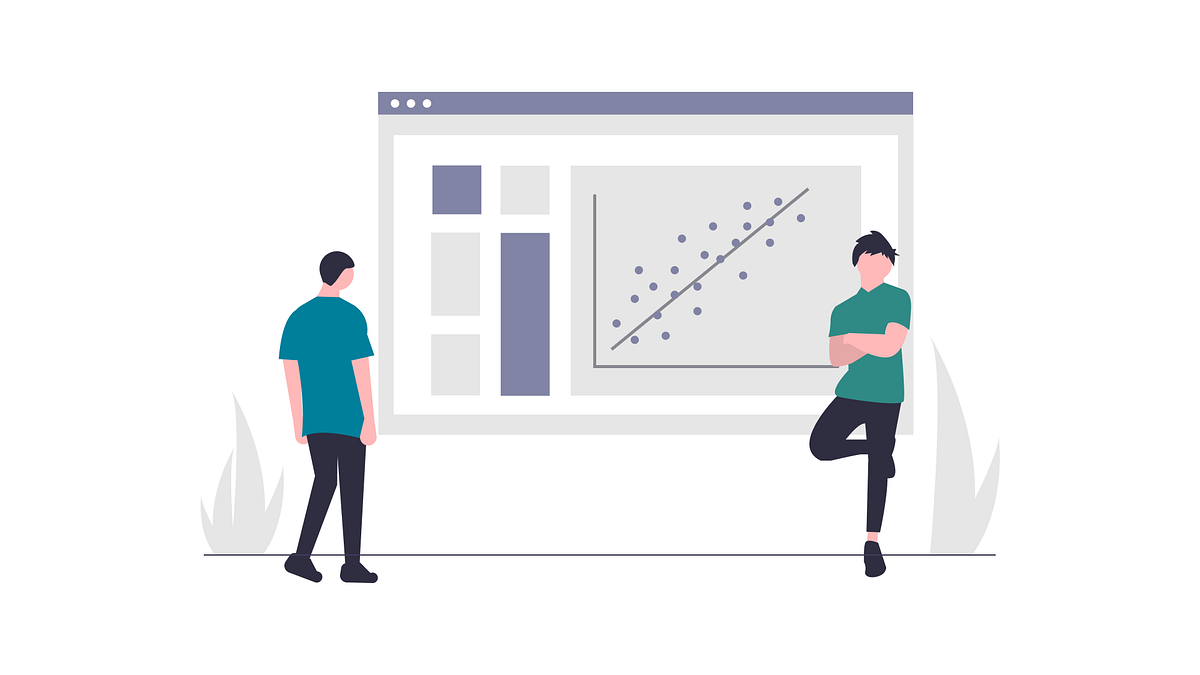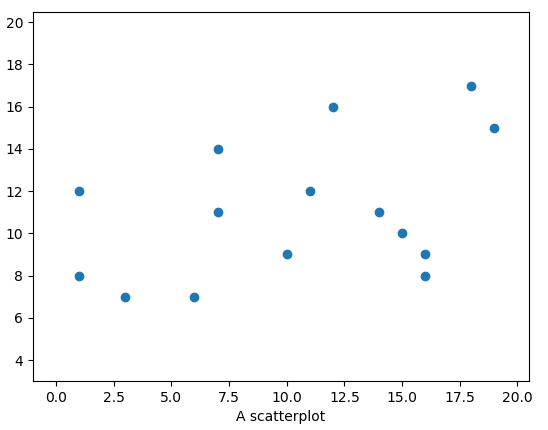# The Linear Regression Equation in a NutshellRegression Analysis is about predicting a value or attribute of a variable based on some other variables. And linear regression is when there is only one variable you want to predict based on another single variable.

What really is regression? Regression Analysis is about predicting a value or attribute of a variable based on some other variables. And linear regression is when there is only one variable you want to predict based on another single variable.

## The Definition

Actually, the formal definition of simple linear regression that I learned at school is, by regression of a variable y on another variable x, we mean the dependence of y on x, on the average. This is more of the answer you can write in your exam sheet. The concept of regression is actually a lot easier than that.

Regression is actually about finding the trend of a data set plotted in a graph. If you draw a regression line ( buzzword alert! ) through a scatter plot, by just looking at the line you can tell if the trend is increasing or decreasing with respect to something!!A matplotlib scatterplot | Image by author

*You should know that regression analysis is the way of calculating and formulating the equation of the line ( do not worry we will get to it ) while the regression line is the line itself. While the equation of simple regression is the *equation of a line.

Y = mX + b

## Intuition

While going around the internet you will find two types of an intuitive approach to linear regression. One is where people will tell you regression is the way you can predict a value of a variable say, y with an input of x which you may already have and that is all right! ( That is the best approach of ML)

The other approach is what I defined in the definition. Think about it, you can draw like thousands of lines through a scatterplot but the line which fits the best and is unique from all every other line is the regression line. The telling it out is in the next paragraph!

## Exploratory Data Analysis is a significant part of Data Science

Data science is omnipresent to advanced statistical and machine learning methods. For whatever length of time that there is data to analyse, the need to investigate is obvious.

## 50 Data Science Jobs That Opened Just Last Week

Data Science and Analytics market evolves to adapt to the constantly changing economic and business environments. Our latest survey report suggests that as the overall Data Science and Analytics market evolves to adapt to the constantly changing economic and business environments, data scientists and AI practitioners should be aware of the skills and tools that the broader community is working on. A good grip in these skills will further help data science enthusiasts to get the best jobs that various industries in their data science functions are offering.

## Learn Data Science using CRISP-DM Framework

If you’re interested in the exciting world of data science, but don’t know where to start, CRISP-DM Framework is here to help.

## Statistical Tests for Data Analysis Part-I

These statistical tests allow researchers to make inferences because they can show whether an observed pattern is due to intervention or chance. There is a wide range of statistical tests.

## Statistics for Data Science Part 1: Use of Central Tendency for Data Analysis.

Explaining the working of the most common central methods like mean, median, mode and how it can help in dealing with our data.As we know to deal with our data has a number of steps like data extraction, data cleaning, handling missing data, exploratory data analysis, etc. and statistics play a very important role in many of these steps